## COMMON I.C.'S PIN ASSIGNMENTS AND LOGIC

### 74175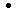74LS17574F175 QUAD D-TYPE FLIP-FLOP

 PIN ASSIGNMENT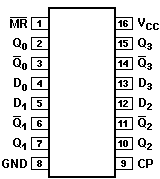LOGIC DIAGRAM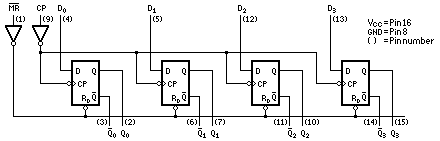TRUTH TABLE

OPERATING MODE INPUTS OUTPUTSMR
CP Dn QnQn
Reset (clear)
Load "1"
Load "0"
L
H
H
X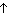X
h
l
L
H
L
H
L
H
` `
H = HIGH voltage level steady state.
h = HIGH voltage level one setup time prior to the LOW-to-HIGH clock transition.
L = LOW voltage level steady state.
l = LOW voltage level one setup time prior to the LOW-to-HIGH clock transition.= LOW-to-HIGH clock transition.
X = don't care.

### 74LS24174F241 OCTAL BUFFER, TRI-STATE

PIN ASSIGNMENT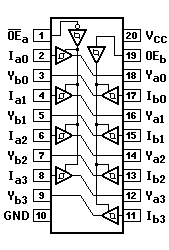LOGIC DIAGRAM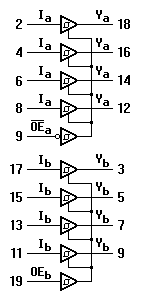TRUTH TABLE

INPUTS OUTPUTSOEa
Ia OEb Ib Ya Yb
L
L
H
L
H
X
H
H
L
L
H
X
L
H
(Z)
L
H
(Z)

H = HIGH voltage level
L = LOW voltage level
X = Don't care
(Z) = HIGH impedance (off) state

### 74LS266 QUAD 2-INPUT EXCLUSIVE NOR GATE (OPEN COLLECTOR)

PIN ASSIGNMENT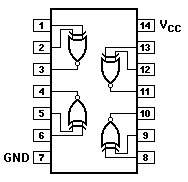LOGIC DIAGRAM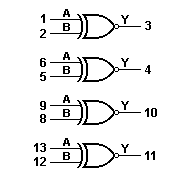TRUTH TABLE

INPUTS OUTPUT
A B Y
L
L
H
H
L
H
L
H
H
L
L
H

H = HIGH voltage level
L = LOW voltage level